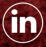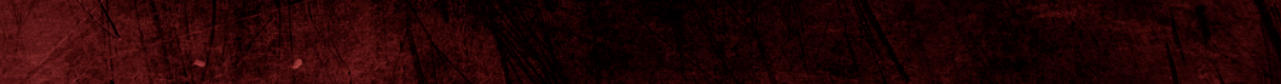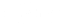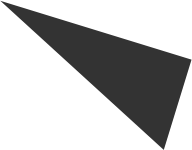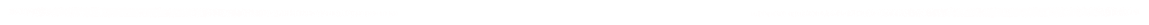L A T E S T    F A I T H   G R A N G E R    N E W S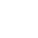W E L C O M E    T O    T H E    F A I T H    G R A N G E R    H U B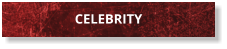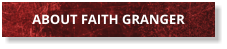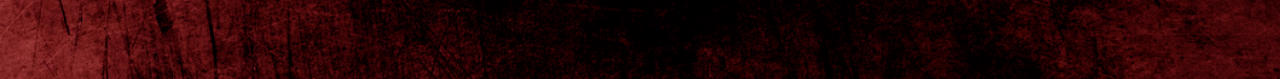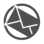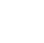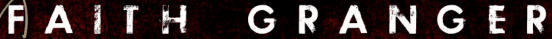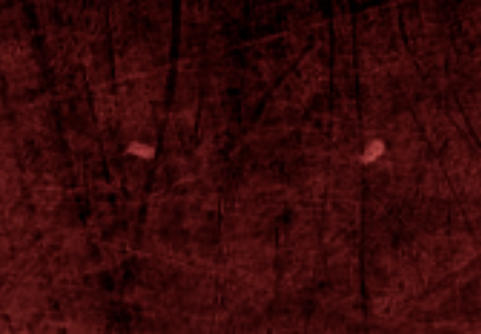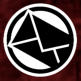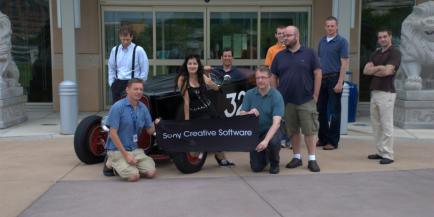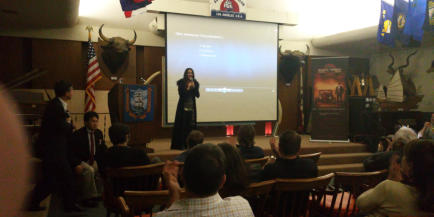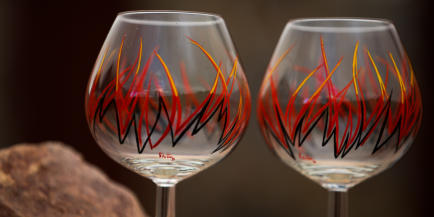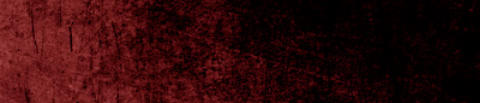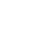L A T E S T   N E W S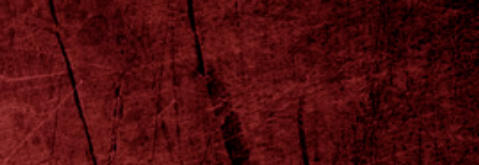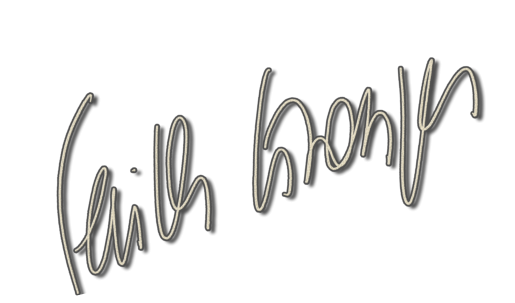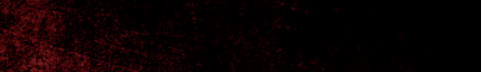W E L C O M E    T O